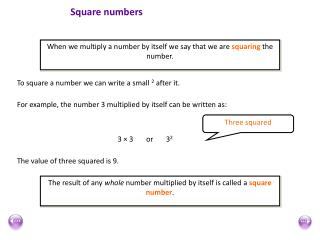Download PresentationSquare numbers

# Square numbers - PowerPoint PPT Presentation

Square numbers. When we multiply a number by itself we say that we are squaring the number. To square a number we can write a small 2 after it. For example, the number 3 multiplied by itself can be written as:. Three squared. 3 × 3 or 3 2. The value of three squared is 9.I am the owner, or an agent authorized to act on behalf of the owner, of the copyrighted work described.
Download Presentation## Square numbers

Download Policy: Content on the Website is provided to you AS IS for your information and personal use and may not be sold / licensed / shared on other websites without getting consent from its author.While downloading, if for some reason you are not able to download a presentation, the publisher may have deleted the file from their server.

- - - - - - - - - - - - - - - - - - - - - - - - - - E N D - - - - - - - - - - - - - - - - - - - - - - - - - -
Presentation Transcript
1. Square numbers When we multiply a number by itself we say that we are squaring the number. To square a number we can write a small 2 after it. For example, the number 3 multiplied by itself can be written as: Three squared 3 × 3 or 32 The value of three squared is 9. The result of any whole number multiplied by itself is called a square number.

2. First 10 square numbers 1 x 1 = 1 2 x 2 = 4 3 x 3 = 9 4 x 4 = 16 5 x 5 = 25 6 x 6 = 36 7 x 7 = 49 8 x 8 = 64 9 x 9 = 81 10 x 10 = 100 First 10 cube numbers 1 x 1 x 1 = 1 2 x 2 x 2 = 8 3 x 3 x 3 = 27 4 x 4 x 4 = 64 5 x 5 x 5 = 125 6 x 6 x 6 = 216 7 x 7 x 7 = 343 8 x 8 x 8 = 512 9 x 9 x 9 = 729 10 x 10 x 10 = 1000 15² = 15 x 15 =225 25² = 25 x 25 = 625 22³ = 22 x 22 x 22 = 10648 15³ = 15 x 15 x 15 = 3375

3. Square roots squared square rooted 64 = Finding the square root is the inverse of finding the square: 8 64 We write: 8 The square root of 64 is 8.

4. Negative square roots 5 × 5 = 25 and –5 ×–5 = 25 Therefore, the square root of 25 is 5 or –5. When we use the  symbol we usually mean the positive square root. We can also write ± to mean both the positive and the negative square root. The equation, x2 = 25 has 2 solutions, or x = 5 x = –5.

5. Square roots 1 = 4 = 9 = 1 or -1 2 or -2 3 or -3 64 = 49 = 36 = 25 = 16 = 81 = 9 or -9 8 or -8 5 or -5 6 or -6 4 or -4 7 or -7 100 = 10 or -10 We can easily find the square root of a square number.

6. Cube roots cubed cube rooted 125 = 5 3 Finding the cube root is the inverse of finding the cube: 5 125 We write: The cube root of 125 is 5.